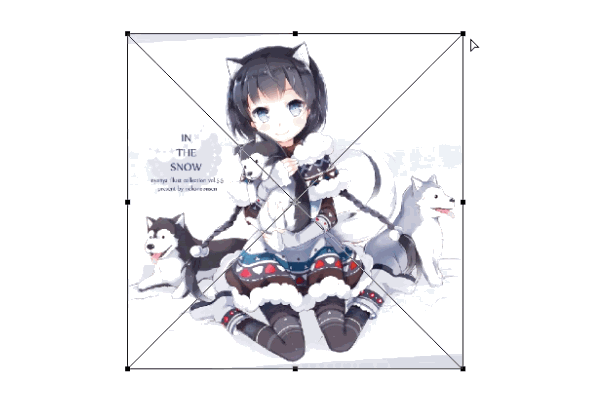# 安卓自定义View进阶-Matrix详解

2022-12-08 13:01:45 michael007js 428

## Matrix方法详解

### 构造方法

#### 无参构造

Matrix ()

Matrix matrix = new Matrix();

#### 有参构造

Matrix (Matrix src)

Matrix matrix = new Matrix(src);

### 数值操作

#### 1.set

void set (Matrix src)

#### 2.reset

void reset ()

#### 3.setValues

void setValues (float[] values)

setValues的参数是浮点型的一维数组，长度需要大于9，拷贝数组中的前9位数值赋值给当前Matrix。

#### 4.getValues

void getValues (float[] values)

### 数值计算

#### 1.mapPoints

void mapPoints (float[] pts)

void mapPoints (float[] dst, float[] src)

void mapPoints (float[] dst, int dstIndex,float[] src, int srcIndex, int pointCount)

(1) void mapPoints (float[] pts) 方法仅有一个参数，pts数组作为参数传递原始数值，计算结果仍存放在pts中。

// 初始数据为三个点 (0, 0) (80, 100) (400, 300)
float[] pts = new float[]{0, 0, 80, 100, 400, 300};

// 构造一个matrix，x坐标缩放0.5
Matrix matrix = new Matrix();
matrix.setScale(0.5f, 1f);

// 输出pts计算之前数据
Log.i(TAG, "before: "+ Arrays.toString(pts));

// 调用map方法计算
matrix.mapPoints(pts);

// 输出pts计算之后数据
Log.i(TAG, "after : "+ Arrays.toString(pts));

before: [0.0, 0.0, 80.0, 100.0, 400.0, 300.0]
after : [0.0, 0.0, 40.0, 100.0, 200.0, 300.0]

(2) void mapPoints (float[] dst, float[] src) ，src作为参数传递原始数值，计算结果存放在dst中，src不变。

// 初始数据为三个点 (0, 0) (80, 100) (400, 300)
float[] src = new float[]{0, 0, 80, 100, 400, 300};
float[] dst = new float;

// 构造一个matrix，x坐标缩放0.5
Matrix matrix = new Matrix();
matrix.setScale(0.5f, 1f);

// 输出计算之前数据
Log.i(TAG, "before: src="+ Arrays.toString(src));
Log.i(TAG, "before: dst="+ Arrays.toString(dst));

// 调用map方法计算
matrix.mapPoints(dst,src);

// 输出计算之后数据
Log.i(TAG, "after : src="+ Arrays.toString(src));
Log.i(TAG, "after : dst="+ Arrays.toString(dst));

before: src=[0.0, 0.0, 80.0, 100.0, 400.0, 300.0]
before: dst=[0.0, 0.0, 0.0, 0.0, 0.0, 0.0]
after : src=[0.0, 0.0, 80.0, 100.0, 400.0, 300.0]
after : dst=[0.0, 0.0, 40.0, 100.0, 200.0, 300.0]

(3) void mapPoints (float[] dst, int dstIndex,float[] src, int srcIndex, int pointCount) 可以指定只计算一部分数值。

// 初始数据为三个点 (0, 0) (80, 100) (400, 300)
float[] src = new float[]{0, 0, 80, 100, 400, 300};
float[] dst = new float;

// 构造一个matrix，x坐标缩放0.5
Matrix matrix = new Matrix();
matrix.setScale(0.5f, 1f);

// 输出计算之前数据
Log.i(TAG, "before: src="+ Arrays.toString(src));
Log.i(TAG, "before: dst="+ Arrays.toString(dst));

// 调用map方法计算(最后一个2表示两个点，即四个数值,并非两个数值)
matrix.mapPoints(dst, 0, src, 2, 2);

// 输出计算之后数据
Log.i(TAG, "after : src="+ Arrays.toString(src));
Log.i(TAG, "after : dst="+ Arrays.toString(dst));

before: src=[0.0, 0.0, 80.0, 100.0, 400.0, 300.0]
before: dst=[0.0, 0.0, 0.0, 0.0, 0.0, 0.0]
after : src=[0.0, 0.0, 80.0, 100.0, 400.0, 300.0]
after : dst=[40.0, 100.0, 200.0, 300.0, 0.0, 0.0]

float mapRadius (float radius)

float radius = 100;
float result = 0;

// 构造一个matrix，x坐标缩放0.5
Matrix matrix = new Matrix();
matrix.setScale(0.5f, 1f);

Log.i(TAG, "mapRadius: "+result);

mapRadius: 100.0
mapRadius: 70.71068

#### 3.mapRect

boolean mapRect (RectF rect)

boolean mapRect (RectF dst, RectF src)

(1) boolean mapRect (RectF rect) 测量rect并将测量结果放入rect中，返回值是判断矩形经过变换后是否仍为矩形。

RectF rect = new RectF(400, 400, 1000, 800);

// 构造一个matrix
Matrix matrix = new Matrix();
matrix.setScale(0.5f, 1f);
matrix.postSkew(1,0);

boolean result = matrix.mapRect(rect);

Log.e(TAG, "isRect: "+ result);

mapRadius: RectF(400.0, 400.0, 1000.0, 800.0)
isRect: false

(2) boolean mapRect (RectF dst, RectF src) 测量src并将测量结果放入dst中，返回值是判断矩形经过变换后是否仍为矩形,和之前没有什么太大区别，此处就不啰嗦了。

#### 4.mapVectors

void mapVectors (float[] vecs)

void mapVectors (float[] dst, float[] src)

void mapVectors (float[] dst, int dstIndex, float[] src, int srcIndex, int vectorCount)

mapVectorsmapPoints 基本上是相同的，可以直接参照上面的mapPoints使用方法。

float[] src = new float[]{1000, 800};
float[] dst = new float;

// 构造一个matrix
Matrix matrix = new Matrix();
matrix.setScale(0.5f, 1f);
matrix.postTranslate(100,100);

// 计算向量, 不受位移影响
matrix.mapVectors(dst, src);
Log.i(TAG, "mapVectors: "+Arrays.toString(dst));

// 计算点
matrix.mapPoints(dst, src);
Log.i(TAG, "mapPoints: "+Arrays.toString(dst));

mapVectors: [500.0, 800.0]
mapPoints: [600.0, 900.0]

### set、pre 与 post

Matrix 相关的重要知识：

• 1.一开始从Canvas中获取到到Matrix并不是初始矩阵，而是经过偏移后到矩阵，且偏移距离就是距离屏幕左上角的位置。

• 这个可以用于判定View在屏幕上的绝对位置，View可以根据所处位置做出调整。

• 2.构造Matrix时使用的是矩阵乘法，前乘(pre)与后乘(post)结果差别很大。

• 这个直接参见上一篇文章 Matrix原理 即可。

• 3.受矩阵乘法影响，后面的执行的操作可能会影响到之前的操作。

• 使用时需要注意构造顺序。

### 特殊方法

#### 1.setPolyToPoly

boolean setPolyToPoly (
float[] src,    // 原始数组 src [x,y]，存储内容为一组点
int srcIndex,   // 原始数组开始位置
float[] dst,    // 目标数组 dst [x,y]，存储内容为一组点
int dstIndex,   // 目标数组开始位置
int pointCount) // 测控点的数量 取值范围是: 0到4

Poly全称是Polygon，多边形的意思，了解了意思大致就能知道这个方法是做什么用的了，应该与PS中自由变换中的扭曲有点类似。public class MatrixSetPolyToPolyTest extends View {

private Bitmap mBitmap;             // 要绘制的图片
private Matrix mPolyMatrix;         // 测试setPolyToPoly用的Matrix

public MatrixSetPolyToPolyTest(Context context) {
super(context);

initBitmapAndMatrix();
}

private void initBitmapAndMatrix() {
mBitmap = BitmapFactory.decodeResource(getResources(),
R.drawable.poly_test);

mPolyMatrix = new Matrix();

float[] src = {0, 0,                                    // 左上
mBitmap.getWidth(), 0,                          // 右上
mBitmap.getWidth(), mBitmap.getHeight(),        // 右下
0, mBitmap.getHeight()};                        // 左下

float[] dst = {0, 0,                                    // 左上
mBitmap.getWidth(), 400,                        // 右上
mBitmap.getWidth(), mBitmap.getHeight() - 200,  // 右下
0, mBitmap.getHeight()};                        // 左下

// 核心要点
mPolyMatrix.setPolyToPoly(src, 0, dst, 0, src.length >> 1); // src.length >> 1 为位移运算 相当于处以2

// 此处为了更好的显示对图片进行了等比缩放和平移(图片本身有点大)
mPolyMatrix.postScale(0.26f, 0.26f);
mPolyMatrix.postTranslate(0,200);
}

@Override
protected void onDraw(Canvas canvas) {
super.onDraw(canvas);

// 根据Matrix绘制一个变换后的图片
canvas.drawBitmap(mBitmap, mPolyMatrix, null);
}
}pointCount为0

pointCount为0和reset是等价的，而不是保持matrix不变，在最底层的实现中可以看到这样的代码：

if (0 == count) {
this->reset();
return true;
}pointCount为1

pointCount为0和translate是等价的，在最底层的实现中可以看到这样的代码：

if (1 == count) {
this->setTranslate(dst.fX - src.fX, dst.fY - src.fY);
return true;
}pointCount为2pointCount为3pointCount为4#### 2.setRectToRect

boolean setRectToRect (RectF src,           // 源区域
RectF dst,                  // 目标区域
Matrix.ScaleToFit stf)      // 缩放适配模式

ScaleToFit 是一个枚举类型，共包含了四种模式:

public class MatrixSetRectToRectTest extends View {

private static final String TAG = "MatrixSetRectToRectTest";

private int mViewWidth, mViewHeight;

private Bitmap mBitmap;             // 要绘制的图片
private Matrix mRectMatrix;         // 测试etRectToRect用的Matrix

public MatrixSetRectToRectTest(Context context) {
super(context);

mBitmap = BitmapFactory.decodeResource(getResources(), R.drawable.rect_test);
mRectMatrix = new Matrix();
}

@Override
protected void onSizeChanged(int w, int h, int oldw, int oldh) {
super.onSizeChanged(w, h, oldw, oldh);
mViewWidth = w;
mViewHeight = h;

}

@Override
protected void onDraw(Canvas canvas) {
super.onDraw(canvas);

RectF src= new RectF(0, 0, mBitmap.getWidth(), mBitmap.getHeight() );
RectF dst = new RectF(0, 0, mViewWidth, mViewHeight );

// 核心要点
mRectMatrix.setRectToRect(src,dst, Matrix.ScaleToFit.CENTER);

// 根据Matrix绘制一个变换后的图片
canvas.drawBitmap(mBitmap, mRectMatrix, new Paint());
}
}#### 4.setSinCos

// 方法一
void setSinCos (float sinValue,     // 旋转角度的sin值
float cosValue)     // 旋转角度的cos值

// 方法二
void setSinCos (float sinValue,     // 旋转角度的sin值
float cosValue,     // 旋转角度的cos值
float px,           // 中心位置x坐标
float py)           // 中心位置y坐标

Matrix matrix = new Matrix();
// 旋转90度
// sin90=1
// cos90=0
matrix.setSinCos(1f, 0f);

Log.i(TAG, "setSinCos:"+matrix.toShortString());

// 重置
matrix.reset();

// 旋转90度
matrix.setRotate(90);

Log.i(TAG, "setRotate:"+matrix.toShortString());

setSinCos:[0.0, -1.0, 0.0][1.0, 0.0, 0.0][0.0, 0.0, 1.0]
setRotate:[0.0, -1.0, 0.0][1.0, 0.0, 0.0][0.0, 0.0, 1.0]

### 矩阵相关

#### 1.invert

boolean invert (Matrix inverse)

Matrix matrix = new Matrix();
Matrix invert = new Matrix();
matrix.setTranslate(200,500);

Log.e(TAG, "before - matrix "+matrix.toShortString() );

Boolean result = matrix.invert(invert);

Log.e(TAG, "after  - result "+result );
Log.e(TAG, "after  - matrix "+matrix.toShortString() );
Log.e(TAG, "after  - invert "+invert.toShortString() );

before - matrix [1.0, 0.0, 200.0][0.0, 1.0, 500.0][0.0, 0.0, 1.0]
after  - result true
after  - matrix [1.0, 0.0, 200.0][0.0, 1.0, 500.0][0.0, 0.0, 1.0]
after  - invert [1.0, 0.0, -200.0][0.0, 1.0, -500.0][0.0, 0.0, 1.0]

#### 2.isAffine

Matrix matrix = new Matrix();
Log.i(TAG,"isAffine="+matrix.isAffine());

matrix.postTranslate(200,0);
matrix.postScale(0.5f, 1);
matrix.postSkew(0,1);
matrix.postRotate(56);

Log.i(TAG,"isAffine="+matrix.isAffine());

isAffine=true
isAffine=true

#### 3.isIdentity

Matrix matrix = new Matrix();
Log.i(TAG,"isIdentity="+matrix.isIdentity());

matrix.postTranslate(200,0);

Log.i(TAG,"isIdentity="+matrix.isIdentity());

isIdentity=true
isIdentity=false

## Matrix实用技巧

### 1.获取View在屏幕上的绝对位置

@Overrideprotected void onDraw(Canvas canvas) {  float[] values = new float;  int[] location1 = new int;  Matrix matrix = canvas.getMatrix();  matrix.getValues(values);  location1 = (int) values;  location1 = (int) values;  Log.i(TAG, "location1 = " + Arrays.toString(location1));  int[] location2 = new int;  this.getLocationOnScreen(location2);  Log.i(TAG, "location2 = " + Arrays.toString(location2));}

location1 = [0, 243]location2 = [0, 243]

### 2.利用setPolyToPoly制造3D效果